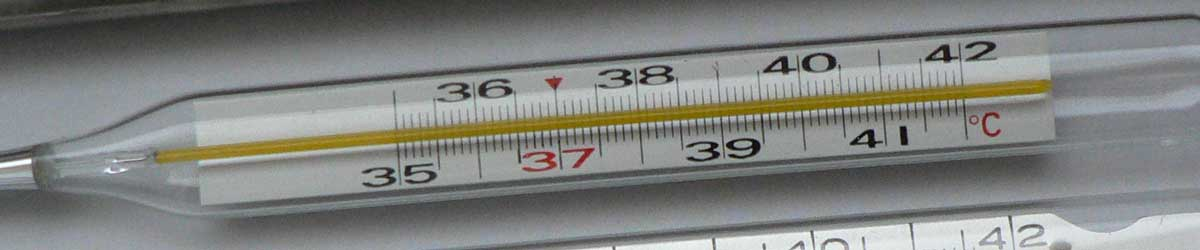# Kelvins to Newton Conversion

Enter the temperature in kelvins below to get the value converted to Newton.

Results in Degrees Newton:1 K = -89.8095 °N
Do you want to convert Newton to kelvins?

## How to Convert Kelvins to NewtonConvert kelvins to degrees Newton with this simple formula:

degrees Newton = ( [K] - 273.15 ) × 33 / 100

Insert the [K] temperature measurement in the formula and then solve to find the result.

For example, let's convert 50 K to degrees Newton:

50 K = ( ( 50 - 273.15 ) × 33 / 100 ) = -73.6395 °N

## Kelvins

The Kelvin scale is an absolute temperature scale that uses absolute zero as the starting point. The kelvin is defined in terms of the Boltzmann constant, which is a measure of the energy of motion corresponding to one kelvin.

More specifically, one kelvin is equal to the change of thermodynamic temperature that results in a change of thermal energy kT by 1.380649 × 10−23 J.

0 kelvins are equal to −273.15 degrees Celsius, which is the point of absolute zero.

The kelvin is the SI base unit for temperature in the metric system. Kelvins can be abbreviated as K; for example, 1 kelvin can be written as 1 K.

The kelvin scale is different from other temperature scales in that it is not expressed in degrees, values are expressed in kelvins. When using the Kelvin scale in writing, the "k" is capitalized, however when using the kelvin as a unit the "k" should be lower-case, even though it is named for a person.

### Important Temperatures In Kelvins

TemperatureKelvins
Absolute Zero0 K
Freezing Point of Water273.15 K
Triple Point of Water273.16 K
Boiling Point of Water373.15 K

## Newton

The Newton scale is a temperature scale that sets a fixed zero point equal to the freezing point water. The scale uses both arithmetic progression and geometric progression. The scale defines 18 reference points and the corresponding "degrees of heat" and geometric progressions.

Newton can be abbreviated as N; for example, 1 degree Newton can be written as 1 °N.

## Kelvin to Newton Conversion Table

Kelvin measurements converted to Newton
Kelvins Newton
0 K -90.14 °N
10 K -86.84 °N
20 K -83.54 °N
30 K -80.24 °N
40 K -76.94 °N
50 K -73.64 °N
60 K -70.34 °N
70 K -67.04 °N
80 K -63.74 °N
90 K -60.44 °N
100 K -57.14 °N
110 K -53.84 °N
120 K -50.54 °N
130 K -47.24 °N
140 K -43.94 °N
150 K -40.64 °N
160 K -37.34 °N
170 K -34.04 °N
180 K -30.74 °N
190 K -27.44 °N
200 K -24.14 °N
273.15 K 0 °N
300 K 8.8605 °N
400 K 41.86 °N
500 K 74.86 °N
600 K 107.86 °N
700 K 140.86 °N
800 K 173.86 °N
900 K 206.86 °N
1,000 K 239.86 °N

## References

1. International Bureau of Weights and Measures, The International System of Units, 9th Edition, 2019, https://www.bipm.org/utils/common/pdf/si-brochure/SI-Brochure-9.pdf